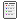###Autor Tema: D_B_X_II_1_11  (Leído 818 veces)

#### Fl0ppy##### D_B_X_II_1_11
« en: 18 de Diciembre de 2018, 08:57:09 pm »Siempre que pasa igual sucede lo mismo#### Fl0ppy##### Re:D_B_X_II_1_11
« Respuesta #1 en: 19 de Diciembre de 2018, 12:42:42 pm »

Quote (selected)
Kernel Time  =     0.218 = 00:00:00.218 =   0%
User Time    =     0.015 = 00:00:00.015 =   0%
Process Time =     0.234 = 00:00:00.234 =   0%
Global Time  = 50376.077 = 13:59:36.077* = 100%(*)horas
Siempre que pasa igual sucede lo mismo#### marc731116##### Re:D_B_X_II_1_11
« Respuesta #2 en: 19 de Diciembre de 2018, 08:11:29 pm »

Quote (selected)
Kernel Time  =     0.218 = 00:00:00.218 =   0%
User Time    =     0.015 = 00:00:00.015 =   0%
Process Time =     0.234 = 00:00:00.234 =   0%
Global Time  = 50376.077 = 13:59:36.077* = 100%(*)horas

14 horas, puffff que es algo de tiempo

#### Fl0ppy##### Re:D_B_X_II_1_11
« Respuesta #3 en: 19 de Diciembre de 2018, 09:14:57 pm »
14 horas

Esto es lo que ha tardado para la compresión
Siempre que pasa igual sucede lo mismo#### Fl0ppy##### Re:D_B_X_II_1_11
« Respuesta #4 en: 23 de Diciembre de 2018, 07:11:06 pm »
Pos ya lo teneis
Siempre que pasa igual sucede lo mismo